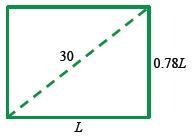### Home > PC3 > Chapter 10 > Lesson 10.2.2 > Problem10-140

10-140.

What are the dimensions of a rectangle whose width is $78\%$ of its length and has a diagonal of length $30$ cm?

Draw and label a diagram.Write and solve an equation using the Pythagorean Theorem.